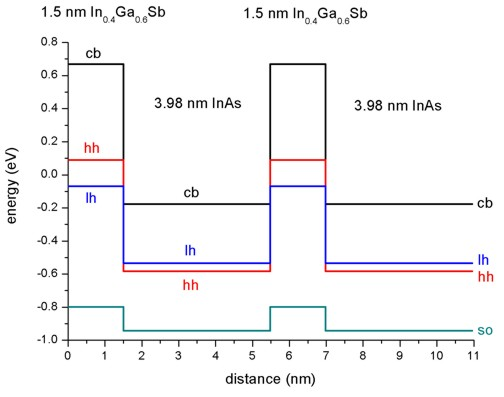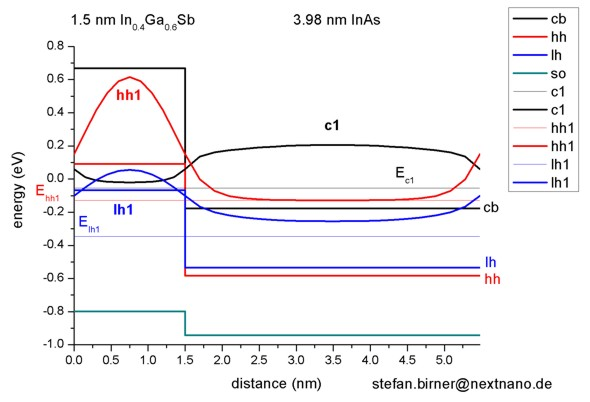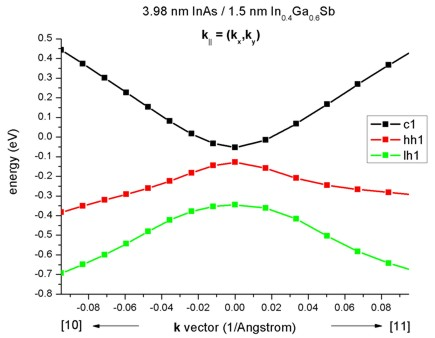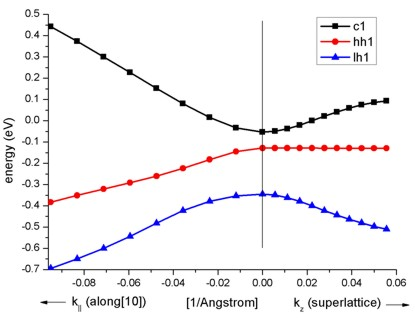nextnano.com  GUI: nextnanomat  Tool: nextnano++  Tool: nextnano³  Tool: nextnano.NEGF  Download | Search | Copyright | News | Publications  * password protected nextnano³ software1D InAs/InGaSb SL (type-II)

# nextnano3 - Tutorial

## InAs / In0.4Ga0.6Sb superlattice dispersion with 8-band k.p (type-II band alignment)

Author: Stefan Birner, Michael Povolotskyi

If you want to obtain the input files that are used within this tutorial, please contact stefan.birner@nextnano.de.
```-> 1DInAs_InGaSb_k_zero_nn3.in                       / *_nnp.in - ```input file for the nextnano3 and nextnano++ software``` -> 1DInAs_InGaSb_k_parallel_nn3.in                              - ```input file for the nextnano3 software (corresponding nextnano++ input file: `1DInAs_InGaSb_k_parallel_k_superlattice_nnp.in`)``` -> 1DInAs_InGaSb_k_superlattice_nn3.in                          - ```input file for the nextnano3 software (corresponding nextnano++ input file: `1DInAs_InGaSb_k_parallel_k_superlattice_nnp.in`)``` -> 1DInAs_InGaSb_SL_k_parallel_k_superlattice_nn3.in / *_nnp.in - ```input file for the nextnano3 and nextnano++ software

## InAs / In0.4Ga0.6Sb superlattice dispersion with 8-band k.p (type-II band alignment)

This tutorial aims to reproduce Fig. 2(a) of

Long wavelength InAs/InGaSb infrared detectors: Optimization of carrier lifetimes
C.H. Grein, P.M. Young, M.E. Flatte, H. Ehrenreich
J. Appl. Phys. 78 (12), 7143 (1995)

### Conduction and valence band edges

`-> 1DInAs_InGaSb_k_zero_nn3.in`

The heterostructure is a superlattice with 3.98 nm InAs and 1.5 nm In0.4Ga0.6Sb, where both constituents are strained with respect to the GaSb substrate.

The structure has a type-II band alignment, i.e. the electrons are confined in the InAs layer,
whereas the holes are confined in the In0.4Ga0.6Sb layer.

The In0.4Ga0.6Sb layer is strained pseudomorphically with respect to the GaSb substrate,
leading to a compressive strain (`-`2.5 %) which splits the degeneracy of the heavy and light hole band edges in this layer.
Thus, the heavy hole band edge lies above the light hole band edge.

The InAs layer is also strained pseudomorphically with respect to the GaSb substrate,
and is thus under slight biaxial tension (+0.6 %).
The splitting of the hole band edges is the opposite as in InGaSb, i.e. the light hole band edge is above the heavy hole band edge.

The following figure shows the electron and hole band edges.The origin of the energy scale is set to the GaSb valence band edge energy.

### Electron and hole wave function for k|| = 0

• We simulate one period only (i.e. from 0 nm to 5.48 nm) and solve the Schrödinger equation with periodic boundary conditions to mimic an infinite superlattice.

The following figure shows the conduction band edge and the heavy, light and split-off hole valence band edges in this superlattice structure
together with the electron (c1), heavy hole (hh1) and light hole (lh1) energies and wave functions (psi²), calculated within 8-band k.p theory.

One can clearly see that the electron state (c1) is confined in the InAs layer (right part of the figure),
whereas the heavy (hh1) and light hole (lh1) states are confined in the In0.4Ga0.6Sb layer (left part of the figure).We used the same material parameters as given in the above cited paper by Grein et al., apart from the k.p parameters.

### Electron and hole energies for k|| /= 0

• ```-> 1DInAs_InGaSb_k_parallel_nn3.in ```
The following figure shows the E(k||) dispersion of the electron ground state and the two highest hole states along two different directions in (kx,ky) space.This data is contained in this file:``` Schroedinger_kp/par1D_disp_01_00_11_hl_8x8kp_ev_min001_ev_max010.dat```
Note that the band gap is not determined by the bandgap of one individual layer. It is determined by the electron ground state in the InAs layer, and the hole ground state in the InGaSb layer. This means more freedom for band gap engineering.

### Electron and hole energies for kz /= 0

• ```-> 1DInAs_InGaSb_k_superlattice_nn3.in ```
The right part of the following figure shows the E(kz) superlattice dispersion of the electron ground state and the two highest hole states. kz is the superlattice vector between 0 and 1 pi/L where L = 5.48 nm is the length of one superlattice period.
(1 pi/L = 0.0573 1/Angstrom)
This data is contained in this file:``` Schroedinger_kp/8x8kp_dispSL_hl_qc001_evmin001_evmax016.dat```

The left part of the figure shows the E(k||) dispersion along , i.e. from (kx,ky) = (0,0) to (kx,ky) = (-0.1,0) which is shown in the figure above already.One can clearly see that these heterostructure bands are highly nonparabolic.

• The resolution of the kz superlattice vectors is specified here:

``` \$quantum-model-electrons   ...   num-ks-001  = 11      ! ```number of superlattice vectors in  direction```   ...  \$quantum-model-holes   ...   num-ks-001  = 11      ! ```number of superlattice vectors in  direction```  ```
• The number of superlattice vectors to be put out is specified here:```  \$output-kp-data   ...   cb-k-SL-min = 1       ! ```only for superlattices: lower bound for range of superlattice vectors (usually equal to 1)```   cb-k-SL-max = 11      ! ```upper bound (usually equal to` num-ks-001`)```   ...   vb-k-SL-min = 1       ! ```only for superlattices: lower bound for range of superlattice vectors (usually equal to 1)```   vb-k-SL-max = 11      ! ```upper bound (usually equal to` num-ks-001`)

• ```-> 1DInAs_InGaSb_SL_k_parallel_superlattice.in ```This input file generates a 3D plot of the the energy dispersion E(kx,ky,kSL) for each eigenvalue. The files are called
```dispersion_k_parallel_k_SL_ev001.fld ```where` ev001 `indicates eigenvalue number 1.

• Please help us to improve our tutorial! Send comments to``` support [at] nextnano.com```.